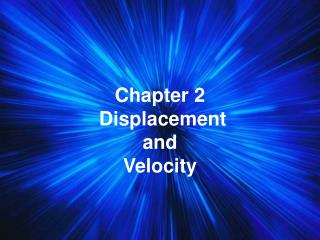DownloadDownload PresentationChapter 2 Displacement and Velocity

Chapter 2 Displacement and Velocity

Download PresentationChapter 2 Displacement and Velocity

- - - - - - - - - - - - - - - - - - - - - - - - - - - E N D - - - - - - - - - - - - - - - - - - - - - - - - - - -
Presentation Transcript

1. Chapter 2 DisplacementandVelocity

2. Frame of Reference - In order to measure the distance of an object we must use a frame of reference. Point A --------> Point B - These are usually distinct points on the Earth's surface or distinguishing points in the sky.

3. The perception of motion depends on the observer’s frame of reference • Describe the motion observed by one of the boys in the drawing, how does the motion appear to be different to the other boy?

4. The perception of motion depends on the observer’s frame of reference • Imagine you are the girl observing the bus, describe the motion of each object that you can see

5. Distance is a scalar quantity and tells you only the magnitude (number sum only) of a path taken.

6. Displacement is the shortest distance between the starting point and the end point.

7. You drive the path, and your odometer goes up by 8 miles (your distance). Your displacement is the shorter directed distance from start to stop (yellow arrow). What if you drove in a circle? Distance vs. Displacement start stop

8. Speed is the distance traveled by a moving object over a period of time

9. Constant speed • A moving object that doesn’t change it’s speed travels at constant speed • Constant speed means equal distances are covered in an equal amount of time

10. Calculating Speed • Speed = Distance Time • If a runner travels 100 m in 10 seconds what was his average speed? • Probably not constant • Can solve for the other pieces too • Distance = speed x time • Time = Distance Speed

11. Velocity is speed in a given direction

12. instantaneous velocity - the velocity that something has at any one instance • (The terms instantaneous speed and avg. speed may also be used)

13. The average velocity for a trip might be 53 miles/hour. However, during this trip your instantaneous speed might have been 0 miles/hour at a stoplight or 70 miles/hour on the open road.